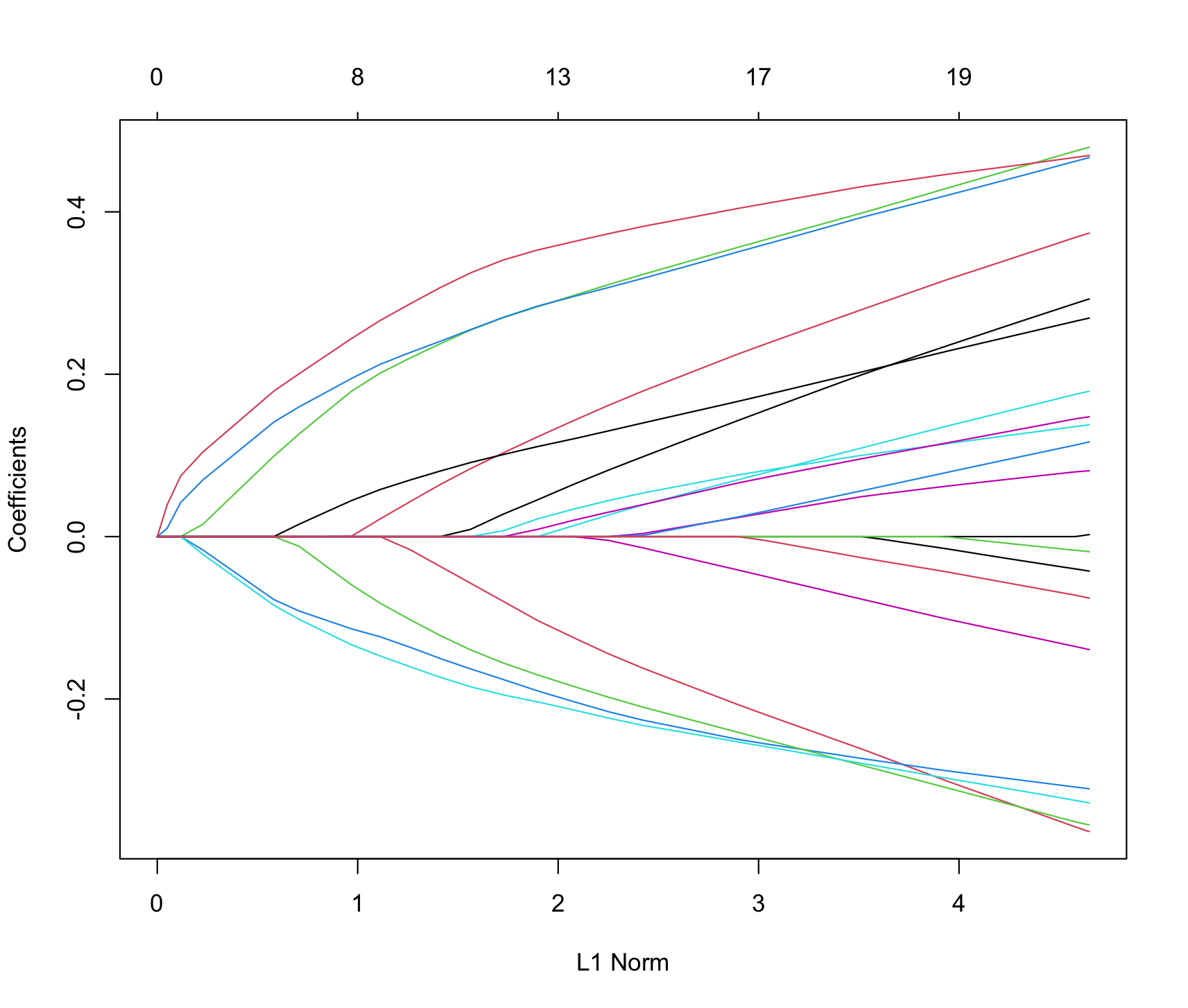# Model Homotopies in the Wild

So are model homotopies commonly used?

Yes, they are.

As an example consider `glmnet`:

Jerome Friedman, Trevor Hastie, Robert Tibshirani (2010). Regularization Paths for Generalized Linear Models via Coordinate Descent. Journal of Statistical Software, 33(1), 1-22. URL http://www.jstatsoft.org/v33/i01/.

From `help(glmnet)`:

```library(glmnet)
x = matrix(rnorm(100 * 20), 100, 20)
g2 = sample(c(0,1), 100, replace = TRUE)
fit2 = glmnet(x, g2, family = "binomial")
```

`fit2` isn’t a model. It is in fact a family of models subscripted by a single variable, in this case by `lambda` the degree of regularization. So it is a model homotopy parameterized by regularization instead of by prevalence.

Further, the `predict(fit2, newx = x)` call returns one prediction for each of these related models, not a prediction from any one model.

This model homotopy even includes a plot method showing the trajectory of the cofficients parameterized by the L1 norm of the coefficients (which themselves are consequences of the regularization trajectory the model homotopy is parameterized by).

```plot(fit2)
```In principle this is a discrete approximation of a fully continuous model homotopy.

Also, in gradient boosting and deep learning, it is common to examine the performance of a family of related models indexed by the training-epoch-number or training-generation-number. In this case the model subscript is discrete, but we see the family of models is reasoned about as a collection. In my opinion this means having a general name for such a collection is of some value.

An example of such a graph is given here:Categories: Opinion Tutorials

Tagged as:### jmount

Data Scientist and trainer at Win Vector LLC. One of the authors of Practical Data Science with R.